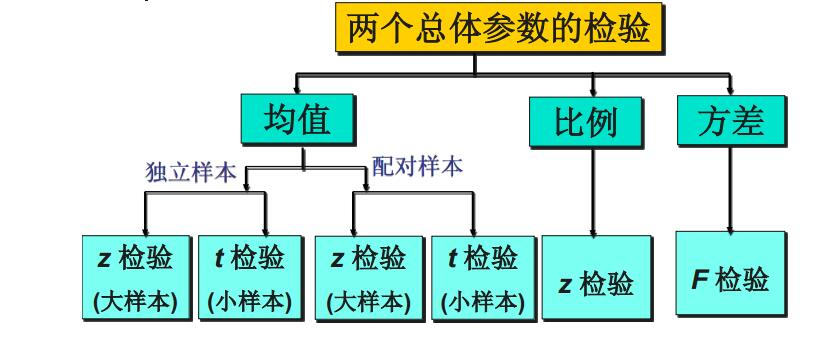# Chapter 6 Hypothesis Test

### 1.基本思想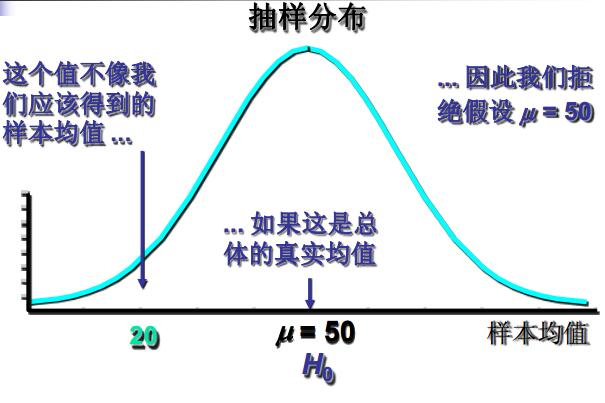http://blog.sciencenet.cn/blog-624263-795715.html

### 2.原假设和备择假设

• 原假设(null hypothesis)——原假设又称“ 0假设”，总是有符号 =， ≥ 或≤，表示为 $H_0$。是研究者想收集证据予以反对的假设（生产实践中常对应正常情形，如均值与设计一致）；一般来说，原假设是一旦拒绝便要采取行动的假设。因此， 原假设总是“受到保护的假设” ，没有充分的证据是不能拒绝原假设的。例如，对一家信誉很好的工厂的产品进行检验，原假设一般是“ 产品合格”。
• 备择假设(alternative hypothesis)——研究者想收集证据予以支持的假设， 一旦发生就要采取行动， 是与原假设对立的假设，也称“研究假设”，总是有符号 ≠， > 或 <，表示为 $H_1$。

• 原假设和备择假设是一个完备事件组， 而且相互对立（在一项假设检验中， 原假设和备择假设必有一个成立， 而且只有一个成立）。
• 先确定备择假设， 再确定原假设。
• 等号“ =” 总是放在原假设上。
• 因研究目的不同， 对同一问题可能提出不同的假设（ 也可能得出不同的结论）。

• 双侧检验——备择假设没有特定的方向性，并含有符号“=”的假设检验，称为双侧检验或双尾检验(two-tailed test)
• 单侧检验——备择假设具有特定的方向性，并含有符号“>”或“<”的假设检验，称为单侧检验或单尾检验(one-tailed test)。其中备择假设的方向为“<”，称为左侧检验，备择假设的方向为“>”，称为右侧检验。

• 双边检验：$H_0: \mu=2，H_1: \mu\neq2$。
• 单边检验：左侧检验——$H_0: \mu\ge2，H_1: \mu<2$，右侧检验——$="" h_0:="" \mu\le2，h_1:="" \mu\="">2$。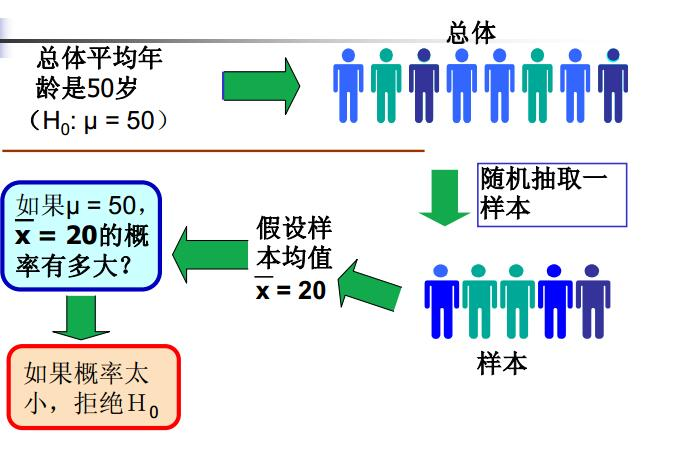• 在一次试验中， 一个几乎不可能发生的事件发生的概率。
• 在一次试验中小概率事件一旦发生， 我们就有理由拒绝原假设。
• 小概率由研究者事先确定。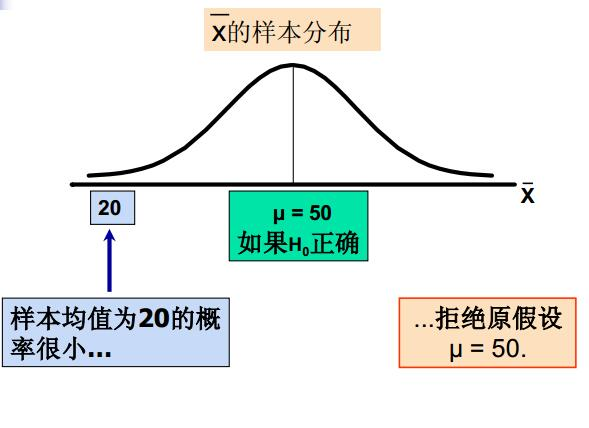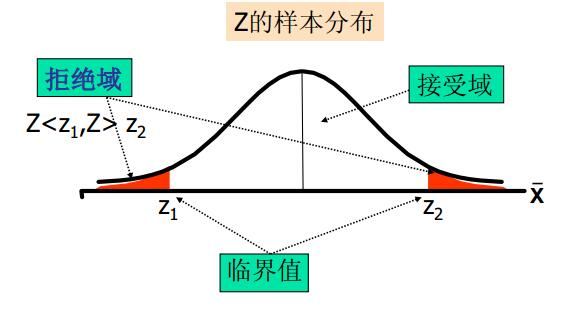### 3.第一类错误和第二类错误

（1）第一类错误(弃真错误)：

• 原假设为真时拒绝原假设。
• 第一类错误的概率为α（没错，就是它，我们的好朋友，小α。咳咳咳，就是显著性水平，一般由研究者事先指定，常用的值有0.01, 0.05, 0.10）。

（2）第二类错误（取伪错误）：

• 原假设为假时未拒绝原假设。
• 第二类错误的概率记为β。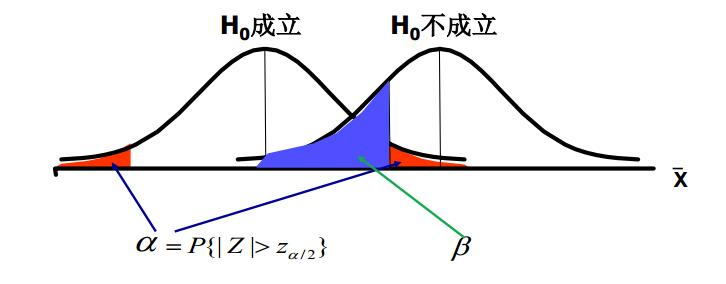α和β的关系——α和β的关系就像翘翘板， α小β就大，α大β就小。所以两类错误不可能同时发生（第一类只在$H_0$为真时发生，第而类只在$H_0$为假时发生）。

• 总体参数的真值。
• 显著性水平α（当α减少时增大）。
• 总体标准差σ（当σ增大时增大）。
• 样本容量n（当n减少时增大）。

### 4.统计量与拒绝域

$$标准化的检验统计量=\frac{点估计量-假设值}{点估计量的抽样标准差}$$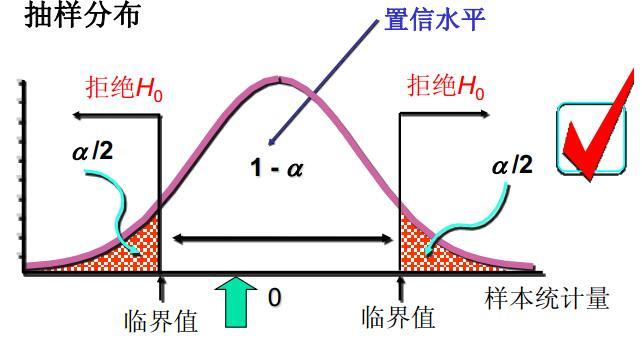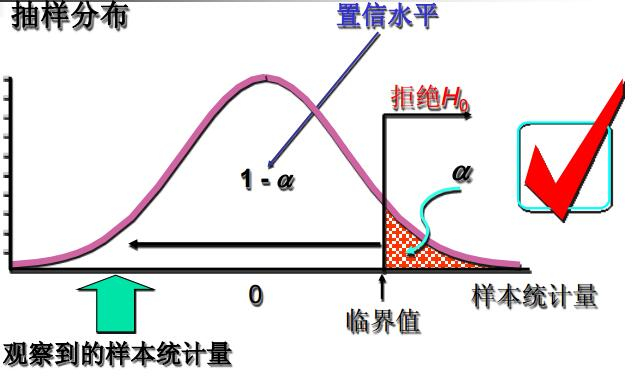• 给定显著性水平α，查表得出相应的临界值$z_{\alpha},z_{\alpha/2},t_{\alpha},t_{\alpha/2},\cdots$。
• 将检验统计量的值与α水平的临界值进行比较。
• 作出决策：双侧检验——|统计量| > 临界值，拒绝$H_0$；左侧检验——统计量 < 临界值，拒绝$H_0$；右侧检验——统计量 > 临界值，拒绝$H_0$。

### 5.利用p值进行决策

p值(p-value)：在一个假设检验问题中，拒绝原假设的最小显著性水平。

• 在原假设为真的条件下，检验统计量的观察值大于或等于其计算值的概率(双侧检验为分布中检验统计量两侧面积的总和;单侧检验为分布中检验统计量相应单侧面积）。
• 反映实际观测到的数据与原假设$H_0$之间的一致程度。
• 被称为观察到的（或实测的）显著性水平。
• 决策规则： 若p值<α， 拒绝$H_0$。

p值法步骤（以大样本均值为例）

• 计算p值： Z为标准正态分布随机变量（$p值=(\left|Z\right|\ge z)(双侧),p值=(Z\le z)(左侧),p值=(Z\ge z)(右侧)$）
• 比较p值和α：
如果α≥p值，拒绝$H_0$;
如果$α<$p值，不能拒绝$H_0$。

• 拒绝原假设时结论是清楚的。
• 当不拒绝原假设时——并未给出明确的结论，不能说原假设是正确的， 也不能说它不是正确的。但也未说它不是10。 我们只能说样本提供的证据还不足以推翻原假设。

• 陈述原假设和备择假设。
• 从所研究的总体中抽出一个随机样本。
• 确定一个适当的检验统计量， 并利用样本数据算出其具体数值。
• 确定一个适当的显著性水平， 并计算出其临界值， 指定拒绝域。
• 将统计量的值与临界值进行比较， 作出决策——统计量的值落在拒绝域，拒绝$H_0$，否则不拒绝$H_0$，也可以直接利用p值作出决策。

### 6.一个总体参数的检验

$\sigma^2已知$：
$$z=\frac{\bar x-\mu_0}{\sigma/\sqrt{n}}\sim N(0,1)。$$
$\sigma^2未知$：
$$z=\frac{\bar x-\mu_0}{s/\sqrt{n}}\sim N(0,1)。$$

$\sigma^2已知$：
$$z=\frac{\bar x-\mu_0}{\sigma/\sqrt{n}}\sim N(0,1)。$$
$\sigma^2未知$：
$$t=\frac{\bar x-\mu_0}{s/\sqrt{n}}\sim t(n-1)。$$

• 总体服从二项分布；
• 可用正态分布来近似(大样本)。

$$z=\frac{\bar q-p_0}{\sqrt{\frac{p_0(1-p_0)}{n}}}\sim N(0,1)，p_0为假设的总体比例。$$

$$\chi^2=\frac{(n-1)s^2}{\sigma_0^2}\sim \chi^2(n-1)。$$• 过程相似：如果假设均值在95%的置信区间之外，双边检验将拒绝原假设（显著性水平为5%）。
• 逻辑不同：置信区间——不知道均值多少而要估计它；假设检验: 假定一个均值要看数据是否支持这个假设。

• α不宜过小，否则第二类错误概率会较大。
• α的选择与判断发生错误时要付出的代价大小有关。
• α的选择是决策问题。

### 7.两个总体参数的检验

• 两个样本是独立的随机样本。
• 大样本($n_1\ge30和n_2\ge30$)。

$$\sigma_1^2，\sigma_2^2已知：z=\frac{(\bar x_1-\bar x_2)-(\mu_1-\mu_2)}{\sqrt{\frac{\sigma_1^2}{n_1}+\frac{\sigma_2^2}{n_2}}}\sim N(0,1)。$$
$$\sigma_1^2，\sigma_2^2未知：z=\frac{(\bar x_1-\bar x_2)-(\mu_1-\mu_2)}{\sqrt{\frac{s_1^2}{n_1}+\frac{s_2^2}{n_2}}}\sim N(0,1)。$$

• 两个独立的小样本。
• 两个总体都是正态分布。
• $\sigma_1^2，\sigma_2^2已知。$

$$z=\frac{(\bar x_1-\bar x_2)-(\mu_1-\mu_2)}{\sqrt{\frac{\sigma_1^2}{n_1}+\frac{\sigma_2^2}{n_2}}}\sim N(0,1)。$$

• 两个独立的小样本。
• 两个总体都是正态分布。
• $\sigma_1^2，\sigma_2^2未知但相等，即\sigma_1^2=\sigma_2^2。$

$$t=\frac{(\bar x_1-\bar x_2)-(\mu_1-\mu_2)}{s_p\sqrt{\frac{1}{n_1}+\frac{1}{n_2}}}，其中s_p=\frac{(n_1-1)s_1^2+(n_2-1)s_2^2}{n_1+n_2-2}，自由度：n_1+n_2-2。$$

• 两个总体都是正态分布。
• $\sigma_1^2，\sigma_2^2未知且不相等，即\sigma_1^2\ne\sigma_2^2。$
• 样本容量相等，$n_1=n_2=n$。

$$t=\frac{(\bar x_1-\bar x_2)-(\mu_1-\mu_2)}{\sqrt{\frac{s_1^2}{n_1}+\frac{s_2^2}{n_2}}}=\frac{(\bar x_1-\bar x_2)-(\mu_1-\mu_2)}{\sqrt{\frac{s_1^2+s_2^2}{n}}}，自由度：n_1+n_2-2=2(n-1)。$$

• 两个总体都是正态分布。
• $\sigma_1^2，\sigma_2^2未知且不相等，即\sigma_1^2\ne\sigma_2^2。$
• 样本容量不相等，$n_1\ne n_2$。

$$t=\frac{(\bar x_1-\bar x_2)-(\mu_1-\mu_2)}{\sqrt{\frac{s_1^2}{n_1}+\frac{s_2^2}{n_2}}}，自由度：最接近v的整数——v=\frac{(\frac{s_1^2}{n_1}+\frac{s_2^2}{n_2})^2}{\frac{(s_1^2/n_1)^2}{n_1-1}+\frac{(s_2^2/n_2)^2}{n_2-1}}。$$

• 两个总体配对差值构成的总体服从正态分布。
• 配对差是由差值总体中随机抽取的。
• 数据配对或匹配(重复测量 (前/后))。

$$样本差值均值\bar d=\frac{\sum_{i=1}^n d_i}{n_d}, 样本差值标准差值 s_d=\sqrt{\frac{\sum_{i=1}^n(d_i-\bar d)^2}{n_d-1}}。$$

$$z=\frac{\bar d-d_0}{s_d/\sqrt{n_d}}\sim N(0,1)。$$

$$t=\frac{\bar d-d_0}{s_d/\sqrt{n_d}}\sim t(n-1)。$$

• 两个总体都服从二项分布。
• 可以用正态分布来近似。

$$z=\frac{\bar p_1-\bar p_2}{\sqrt{\bar p(1-\bar p)(\frac{1}{n_1}+\frac{1}{n_2})}}，其中\bar p=\frac{x_1+x_2}{n_1+n_2}=\frac{\bar p_1n_1+\bar p_2n_2}{n_1+n_2}。$$

$$z=\frac{(\bar p_1-\bar p_2)-d_0}{\sqrt{\frac{\bar p_1(1-\bar p_1)}{n_1}+\frac{\bar p_2(1-\bar p_2)}{n_2}}}。$$

• 两个总体都服从正态分布。
• 两个独立的随机样本。

$$F=\frac{s_1^2}{s_2^2}\sim F(n_1-1,n_2-1)或 F=\frac{s_2^2}{s_1^2}\sim F(n_2-1,n_1-1)。$$